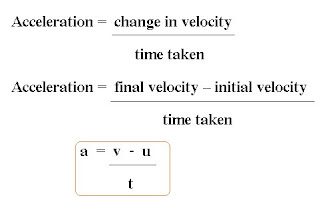## Friday, March 18, 2011

### Acceleration and Deceleration (Retardation)

In the context of linear motion, the direction of the object in motion does not change; but the magnitude of the velocity may change.
When the velocity of an object changes, the object is said to be accelerating.

Acceleration is defined as the rate of change in velocity.Acceleration is a vector quantity. SI unit for acceleration, a is ms-2, metre per second squared.Positive acceleration
If the velocity of an object increases from an initial velocity, u, to a higher final velocity, v.
The acceleration of an object is uniform if its velocity increases at a uniform rate.

Negative acceleration is called deceleration.
If the velocity of an object decreases from an initial velocity, u, to a lower final velocity, v, its acceleration is negative.
The deceleration of an object is uniform if its velocity decreases at a uniform rate.

Zero acceleration
An object moving at a constant velocity, that is, the magnitude and direction of its velocity remain unchanged - is not accelerating; its acceleration is zero.

Quiz :

An object accelerates at a constant rate from rest and reaches a velocity of 10 ms-1 after 5.0 s.
What is it acceleration?

Tips : rest mean stationary ( zero velocity )

## Wednesday, March 2, 2011

### Speed of Sound

The speed of sound, v, in a medium can be determined using the formula v = fλ , whereλ is the wavelength and f is the frequency of the sound wave.

The SI units of v, f and λ are ms-1 , Hz and metre (m) respectively.

The speed of sound in solids is greater than the speed of sound in liquids which is greater than the speed of sound in gases.

The speed of sound is unaffected by pressure. If the atmospheric pressure changes, the speed of sound in air remains constant.

The speed of sound increases with temperature. At the peak of high-altitude mountains, the speed of sound is less than that at sea level. This is not due to the lower pressures but instead it is because of lower temperatures at the peak of the mountain.